## Telco Customer Churn

### 1) About:

Churn rate is a measure of the number of indivisuals or items moving out of a group/organisation over a specific period. Hence it serves as an important metric for companies whose customers pay in a recurrent manner. It helps mostly subscription based companies to have a ballpark estimate of how many customers they will have sticking around over a period of time, visually the line of saturation in the graph developed gradually for a given time period. Note that this equilibrium may vary over years according to company strategies which are essentially ways to lure customers thus altering the churn rate to an ideal low.

### 2) Dataset Overview

Each row represents a customer, each column contains customer’s attributes described on the column metadata.

The data set includes information about:

1. Customers who left within the last month – the column is called Churn

2. Services that each customer has signed up for – phone, multiple lines, internet, online security, online backup, device protection, tech support, and streaming TV and movies

3. Customer account information – how long they’ve been a customer, contract, payment method, paperless billing, monthly charges, and total charges

4. Demographic info about customers – gender, age range, and if they have partners and dependents

### 3) Objective:

To derive better meaning out of the given data by mere observation and visualisation aided comparision

In :
``````#importing lib
import warnings
warnings.filterwarnings("ignore", category=DeprecationWarning)
warnings.filterwarnings("ignore", category=FutureWarning)

import numpy as np
import pandas as pd
import matplotlib.pyplot as plt
import seaborn as sns
import jovian

#importing dataset
dataset = pd.read_csv("WA_Fn-UseC_-Telco-Customer-Churn.csv", index_col='customerID')
dataset['PaymentMethod'] = dataset['PaymentMethod'].replace('Bank transfer (automatic)', 'Bank transfer')
dataset['PaymentMethod'] =dataset['PaymentMethod'].replace('Credit card (automatic)', 'Credit card')

#splitting dataset / churn
bye = dataset[dataset['Churn'] == 'Yes']
nobye = dataset[dataset['Churn'] == 'No']

#fixing missing values
dataset['TotalCharges'] = dataset['TotalCharges'].replace(" ", 0)
temp = dataset['TotalCharges'].values.reshape(-1,1).astype('float64')
from sklearn.preprocessing import Imputer
imputer = Imputer(missing_values=0., strategy='mean', axis = 0)
imputer.fit(temp[:, :])
imputer.transform(temp[:, :])
dataset['TotalCharges'] = temp

#splitting in homogeneous categories
cat_feat = list(dataset.columns)
cat_feat.remove('tenure')
cat_feat.remove('MonthlyCharges')
cat_feat.remove('TotalCharges')
cat_feat.remove('Churn')

num_feat = ['tenure', 'MonthlyCharges', 'TotalCharges']
pred = 'Churn'``````
```/home/shreesh/.local/lib/python3.6/site-packages/sklearn/utils/deprecation.py:66: DeprecationWarning: Class Imputer is deprecated; Imputer was deprecated in version 0.20 and will be removed in 0.22. Import impute.SimpleImputer from sklearn instead. warnings.warn(msg, category=DeprecationWarning) ```

### 4) Exploratory Analysis

#### 4.1) Churn Distribution

In :
``````plt.figure(figsize = (7,6))
plt.title("Churn distribution")
sns.set_style('darkgrid')
plot = sns.countplot(x = 'Churn', data = dataset, palette='Set2')
for p in plot.patches:
plot.annotate('{}'.format(p.get_height()), (p.get_x()+0.3, p.get_height()+50))
plt.ylabel("Number of people", fontsize = 13)
plt.xlabel("Churn", fontsize = 13)
plt.show()
``````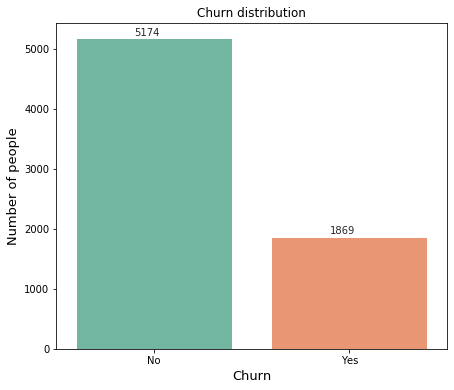#### a) Numerical Analysis

In :
``````total = dataset.shape
left = len(dataset[dataset['Churn']=='Yes'])
stayed = len(dataset[dataset['Churn'] == 'No'])
print("Total: {}\n%Left: {}%\n%Stayed: {}%".format(total, left/total*100, stayed/total*100))``````
```Total: 7043 %Left: 26.536987079369588% %Stayed: 73.4630129206304% ```

#### b) Inference

Referring to Buisness Daily a customer retention rate of 73% is fairly low, perhaps excavating further would help us get to the thicker roots of customer dissatisfaction

### 4.2) Categorical breakdown

In :
``````def analyse(X):
plt.figure(figsize=(9,6))
sns.set_style('darkgrid')
plt.title("{} distribution".format(X.capitalize()), fontsize=18)
plot = sns.countplot(x = "{}".format(X), data=dataset, hue = dataset['Churn'], palette = 'Set2')
plt.ylabel("Number of people", fontsize=14)
plt.xlabel("{}".format(X), fontsize=14)
for p in plot.patches:
plot.annotate('{}'.format(p.get_height()), (p.get_x()+0.1, p.get_height()+4))
plt.show
col = X
total = len(dataset[col])
for cat in dataset[col].unique():
if (col == 'SeniorCitizen'):
q = len(dataset[dataset[col]==cat])
if cat == 0:
print("%Not senior:\nClass distribution: {}% \nRetention rate: {}%".format(round(q/total*100,2),round((1-len(bye[bye[col]==cat])/len(dataset[dataset[col]==cat]))*100,2)))
else:
print("%Senior:\nClass distribution: {}% \nRetention rate: {}%".format(round(q/total*100,2),round((1-len(bye[bye[col]==cat])/len(dataset[dataset[col]==cat]))*100,2)))
else:
q = len(dataset[dataset[col]=='{}'.format(cat)])
print("%{}:\nClass distribution: {}% \nRetention rate: {}%".format(cat, round(q/total*100,2),round((1-len(bye[bye[col]=='{}'.format(cat)])/len(dataset[dataset[col]=='{}'.format(cat)]))*100,2)))``````

#### a) Gender

In :
``analyse('gender')``
```%Female: Class distribution: 49.52% Retention rate: 73.08% %Male: Class distribution: 50.48% Retention rate: 73.84% ```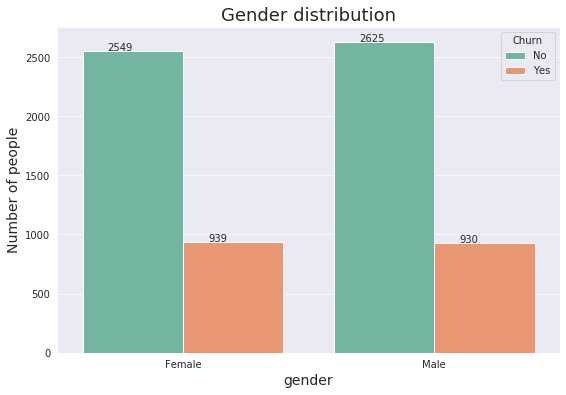``Female customers are more likely to leave vs Male customers, but the difference is almost negligible ~0.04%, thus ruling out gender as a basis of judgement``

#### b) Age group

In :
``analyse('SeniorCitizen')``
```%Not senior: Class distribution: 83.79% Retention rate: 76.39% %Senior: Class distribution: 16.21% Retention rate: 58.32% ```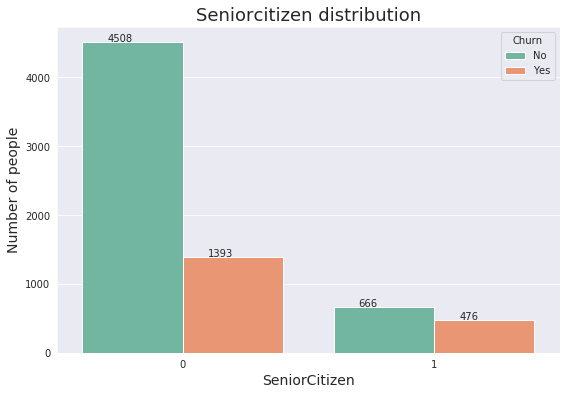``Senior citizens churned a lot more than the younger age groups, yeilding a retention rate of only 58.32% while younger age groups demonstrate a retention rate ~76.39%``

#### c) Partner

In :
``analyse('Partner')``
```%Yes: Class distribution: 48.3% Retention rate: 80.34% %No: Class distribution: 51.7% Retention rate: 67.04% ```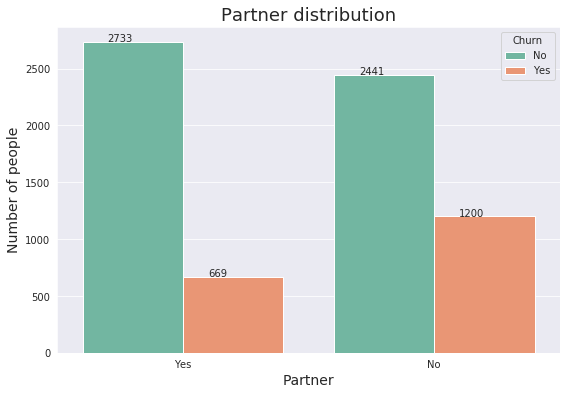#### d) Dependendent

In :
``analyse('Dependents')``
```%No: Class distribution: 70.04% Retention rate: 68.72% %Yes: Class distribution: 29.96% Retention rate: 84.55% ```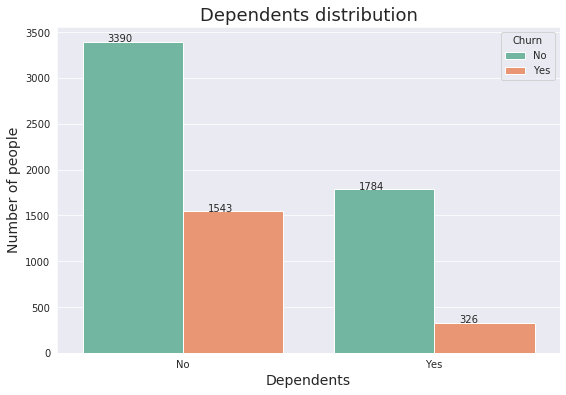``Coincidentally dependent groups and couples seemed to have a bad experience with the company hence contributing identically to the churn``

#### d) Phone Service

In :
``analyse("PhoneService")``
```%No: Class distribution: 9.68% Retention rate: 75.07% %Yes: Class distribution: 90.32% Retention rate: 73.29% ```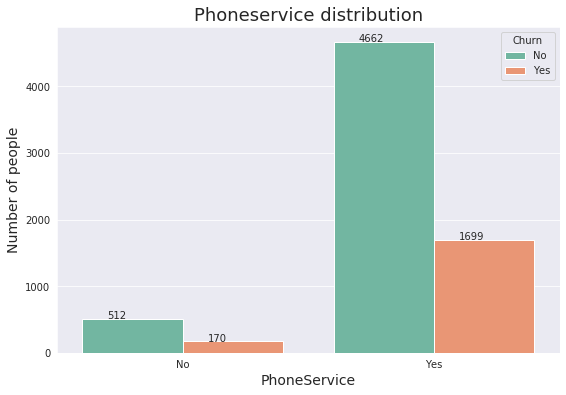``About 90% users opted for the phone services of which almost 3/4th continued their subscription indicating customer satisfaction in that domain``

#### e) Multiple Line Service

In :
``analyse('MultipleLines')``
```%No phone service: Class distribution: 9.68% Retention rate: 75.07% %No: Class distribution: 48.13% Retention rate: 74.96% %Yes: Class distribution: 42.18% Retention rate: 71.39% ```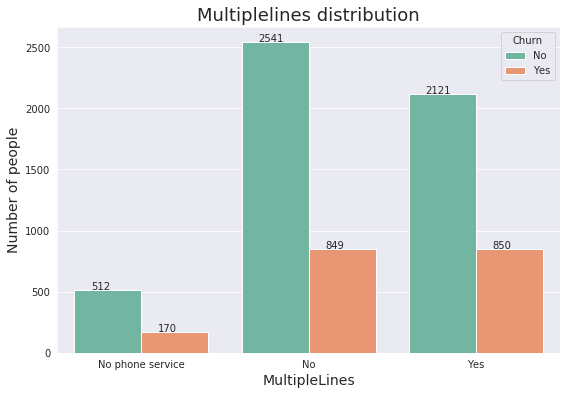``Almost equal number of customer opted for multiple line services. The stats recorded an identical retention rate for both halves thus indicating satisfactory multiple line services offered by the company``

#### f) Internet Services

In :
``analyse('InternetService')``
```%DSL: Class distribution: 34.37% Retention rate: 81.04% %Fiber optic: Class distribution: 43.96% Retention rate: 58.11% %No: Class distribution: 21.67% Retention rate: 92.6% ```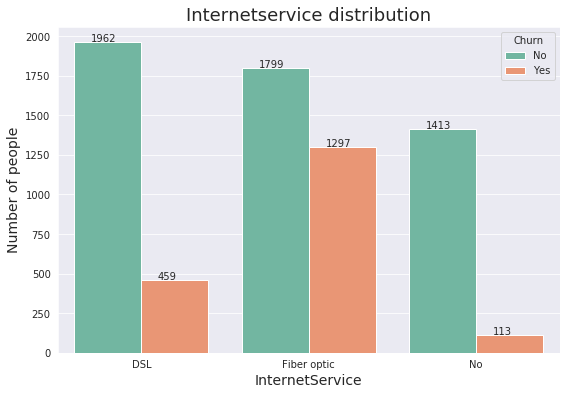``Contrary to expectations, fiber optic services, despite being the faster of the two internet services churned at an alarming rate``

#### g) Addon Services

In :
``````print("Online Security")
analyse('OnlineSecurity')
print('---------------------------\n\n')
print("Online Backup")
analyse('OnlineBackup')
print('---------------------------\n\n')
print("Device Protection")
analyse('DeviceProtection')
print('---------------------------\n\n')
print('Tech Support')
analyse('TechSupport')``````
```Online Security %No: Class distribution: 49.67% Retention rate: 58.23% %Yes: Class distribution: 28.67% Retention rate: 85.39% %No internet service: Class distribution: 21.67% Retention rate: 92.6% --------------------------- Online Backup %Yes: Class distribution: 34.49% Retention rate: 78.47% %No: Class distribution: 43.84% Retention rate: 60.07% %No internet service: Class distribution: 21.67% Retention rate: 92.6% --------------------------- Device Protection %No: Class distribution: 43.94% Retention rate: 60.87% %Yes: Class distribution: 34.39% Retention rate: 77.5% %No internet service: Class distribution: 21.67% Retention rate: 92.6% --------------------------- Tech Support %No: Class distribution: 49.31% Retention rate: 58.36% %Yes: Class distribution: 29.02% Retention rate: 84.83% %No internet service: Class distribution: 21.67% Retention rate: 92.6% ```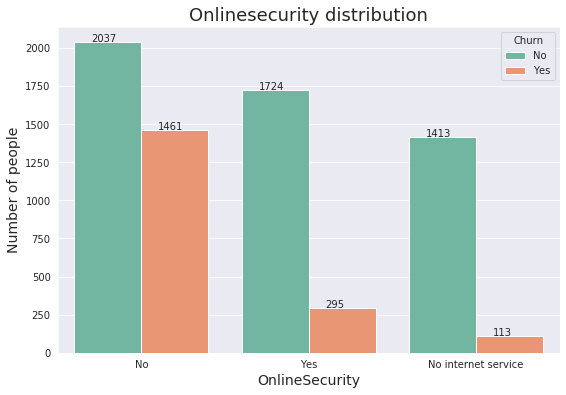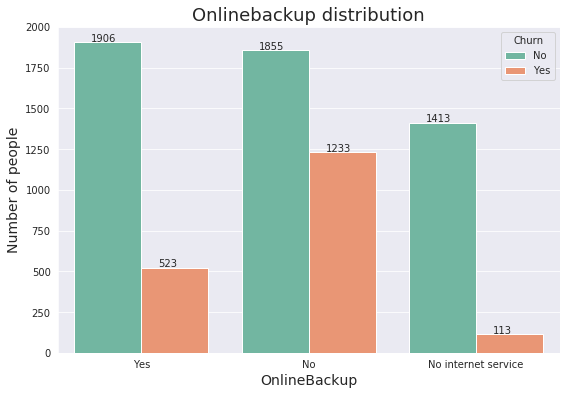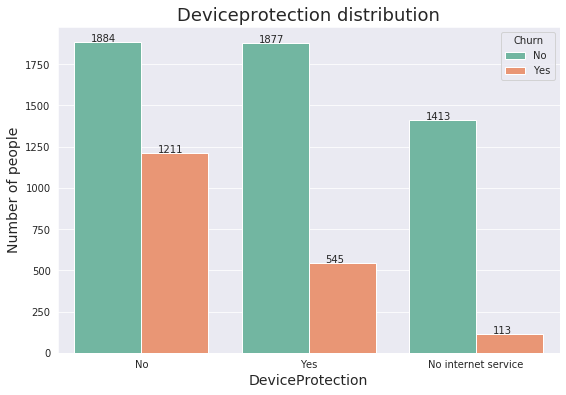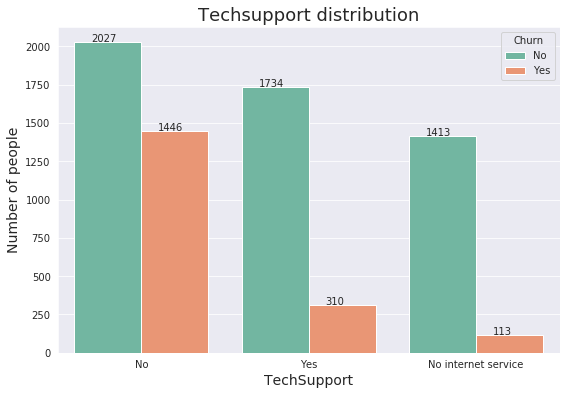``People who did not purchase services like online security, online backup and device protection churned more than the ones who did. ``
In :
``````onsec = dataset[dataset['OnlineSecurity']=='Yes']
onbac = dataset[dataset['OnlineBackup']=='Yes']
dp = dataset[dataset['DeviceProtection']=='Yes']
tech = dataset[dataset['TechSupport']=='Yes']
no_tech = dataset[dataset['TechSupport']=='No']
services_w_support=pd.concat([onbac,onsec, dp], ignore_index=True).drop_duplicates()
services_w_support=pd.merge(services_w_support, tech, how='inner')
services_wo_support = pd.concat([onbac, onsec, dp])
services_wo_support = pd.merge(services_wo_support, no_tech, how='inner')
churn_w = len(services_w_support[services_w_support['Churn']=='Yes'])
churn_wo = len(services_wo_support[services_wo_support['Churn']=='Yes'])
print("Churn rate")
print("% People w service but wo support: {}%".format(churn_wo/(churn_wo+churn_w)*100))
print("% People w service but w support: {}%".format(churn_w/(churn_wo+churn_w)*100))``````
```Churn rate % People w service but wo support: 80.83197389885808% % People w service but w support: 19.168026101141926% ```
``Evidently customers who opted for afore mentioned services but didn't go for helping services like tech support dominated the attrition rate <br> <br>``

#### h) Streaming Services

In :
``````print("Streaming TV")
analyse('StreamingTV')
print("--------------------------\n\n")
print('Streaming Movies')
analyse('StreamingMovies')
print("--------------------------\n\n")``````
```Streaming TV %No: Class distribution: 39.9% Retention rate: 66.48% %Yes: Class distribution: 38.44% Retention rate: 69.93% %No internet service: Class distribution: 21.67% Retention rate: 92.6% -------------------------- Streaming Movies %No: Class distribution: 39.54% Retention rate: 66.32% %Yes: Class distribution: 38.79% Retention rate: 70.06% %No internet service: Class distribution: 21.67% Retention rate: 92.6% -------------------------- ```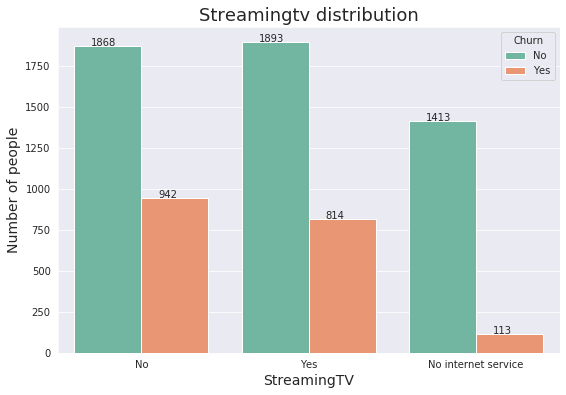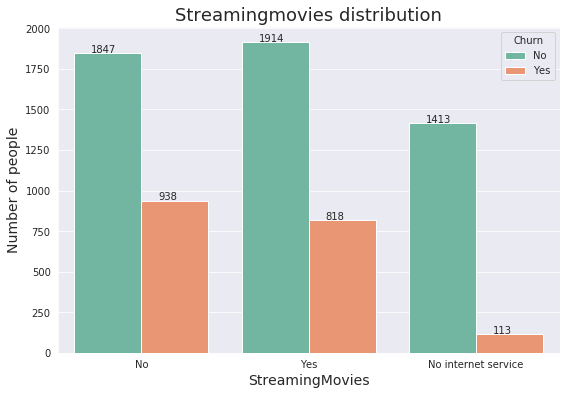In :
``````telecom_churn_services = dataset[['OnlineSecurity', 'DeviceProtection', 'StreamingMovies'
,'TechSupport', 'StreamingTV', 'OnlineBackup', 'Churn']]
telecom_churn_services.replace(to_replace='Yes', value=1, inplace=True)
telecom_churn_services.replace(to_replace='No', value=0, inplace=True)
telecom_churn_services = telecom_churn_services[telecom_churn_services.OnlineSecurity !='No internet service']
all_services = telecom_churn_services.groupby('Churn', as_index=False)[['OnlineSecurity', 'DeviceProtection', 'StreamingMovies', 'TechSupport', 'StreamingTV', 'OnlineBackup']].sum()``````
```/home/shreesh/.local/lib/python3.6/site-packages/pandas/core/frame.py:4263: SettingWithCopyWarning: A value is trying to be set on a copy of a slice from a DataFrame See the caveats in the documentation: http://pandas.pydata.org/pandas-docs/stable/user_guide/indexing.html#returning-a-view-versus-a-copy method=method, ```
In :
``````ax = all_services.set_index('Churn').T.plot(kind='bar', stacked=True, figsize=(12,6))
patches, labels = ax.get_legend_handles_labels()
ax.legend(patches, labels, loc='best')
ax.set_title('Addon Services vs Churn rate', fontsize=20)
plt.show()
``````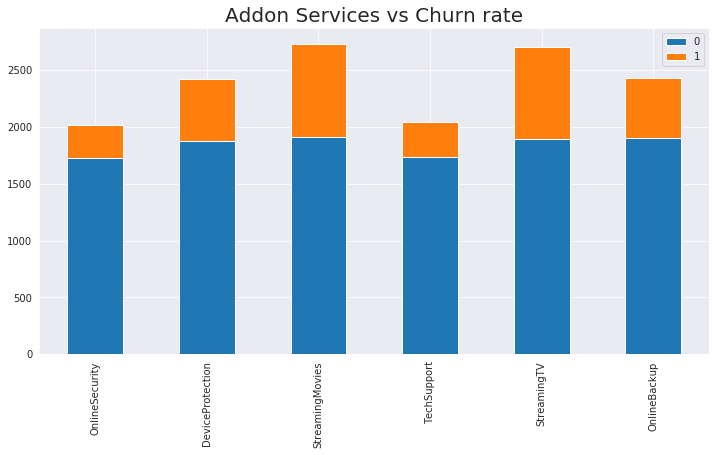``Although the comparision of streaming category and churn class alone doesn't throw much information. But by comparing all the services offered we can tell how Streaming services offered were relatively the poorest of all``

#### i) Payment Method

In :
``````plt.figure(figsize=(10,6))
sns.countplot(x = 'PaymentMethod', data=bye, palette='Set2')
plt.show()
``````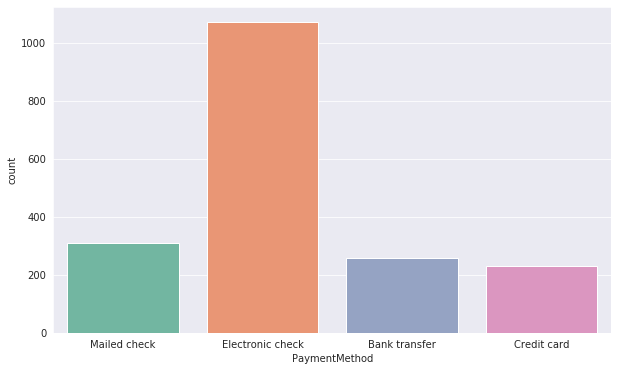``Wouldn't take a genius to figure out how bad their interface for electronic check payement has been. A devastatingly low retention rate of 54.71%``

#### j) Contract

In :
``analyse('Contract')``
```%Month-to-month: Class distribution: 55.02% Retention rate: 57.29% %One year: Class distribution: 20.91% Retention rate: 88.73% %Two year: Class distribution: 24.07% Retention rate: 97.17% ```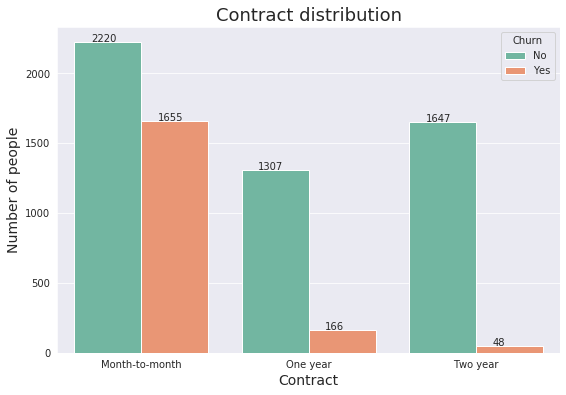``Customers with a shorter contract were observed to churn often as compared to the ones w a contract ~2 years who barely churned``

### 3. Numeric Feature Breakdown

In :
``dataset[num_feat].describe().transpose()``
Out:
``The evident skewness in the the frequency distribution of numerical features reflect their relation with the variable of concern (churn). We notice that greater Total Charges and Tenure were correspond to a lower churn rate, thus favoring the company``

#### a) Charges - Overview

In :
``````fig, ax = plt.subplots(1, 3, figsize=(16, 5))
dataset[dataset.Churn == "No"][num_feat].hist(bins=30, color="lime", alpha=0.7, ax=ax)
dataset[dataset.Churn == "Yes"][num_feat].hist(bins=30, color="black", alpha=0.6, ax=ax)
plt.show()
``````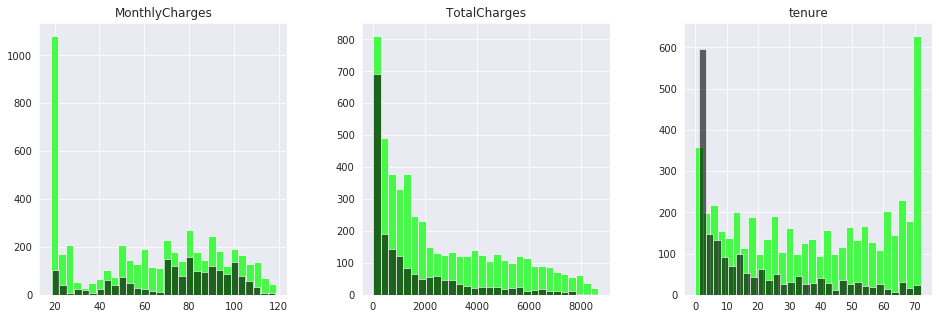#### b) Tenure and Total Charges

In :
``````plt.figure(figsize = (10,5))
plt.title("KDE-Plot: Tenure")
sns.kdeplot(dataset[dataset['Churn']=='Yes']['tenure'], color = 'orange', label = 'Tenure - Churned')
sns.kdeplot(dataset[dataset['Churn']=='No']['tenure'], color = 'blue', label = 'Tenure - Stayed')
plt.show()

plt.figure(figsize = (10,5))
plt.title("KDE-Plot: Total Charges")
sns.kdeplot(dataset[dataset['Churn']=='Yes']['TotalCharges'], color = 'orange', label = 'Total -Churned')
sns.kdeplot(dataset[dataset['Churn']=='No']['TotalCharges'], color = 'blue', label = 'Total -Stayed')
plt.show()

plt.figure(figsize=(10, 5))
sns.heatmap(dataset.corr(),
xticklabels=dataset.corr().columns,
yticklabels=dataset.corr().columns,
cmap="YlGnBu")
plt.title('Correlation Heatmap', fontsize=15)
plt.show()
``````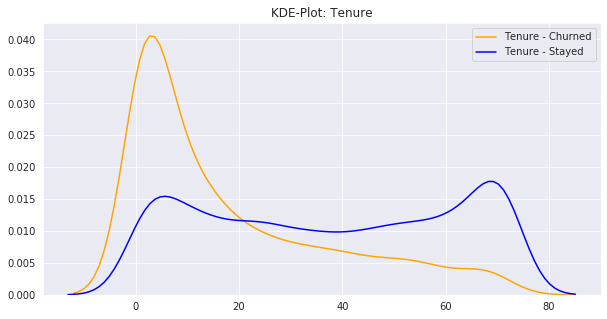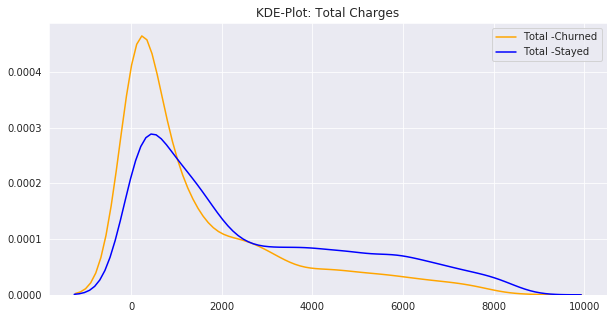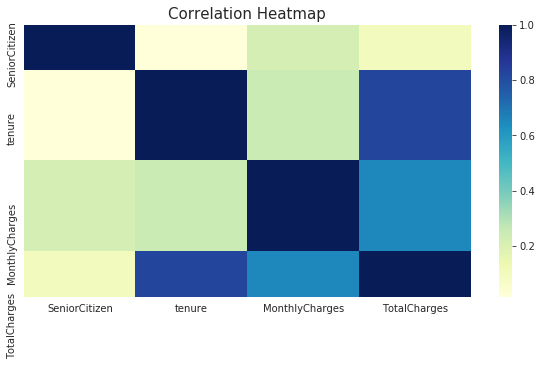``It is the strong correlation between tenure and total charges that justifies the weird density distribution of total cahrges. Simply because customers stayed longer they ended up having a higher bill towards the end. This does not imply that customers with higher total charges stuck around more than the counterpart. Mere surface analysis says otherwise``

#### c) Monthly Charges

In :
``````plt.figure(figsize=(16,6))
sns.kdeplot(dataset[dataset['Churn']=='Yes']['MonthlyCharges'], color = 'blue', label = 'Churned')
sns.kdeplot(dataset[dataset['Churn']=='No']['MonthlyCharges'], color = 'orange', label = 'Stayed')
plt.title('Frequency Distribution of Monthly Charges', fontsize = 16)
plt.show()
``````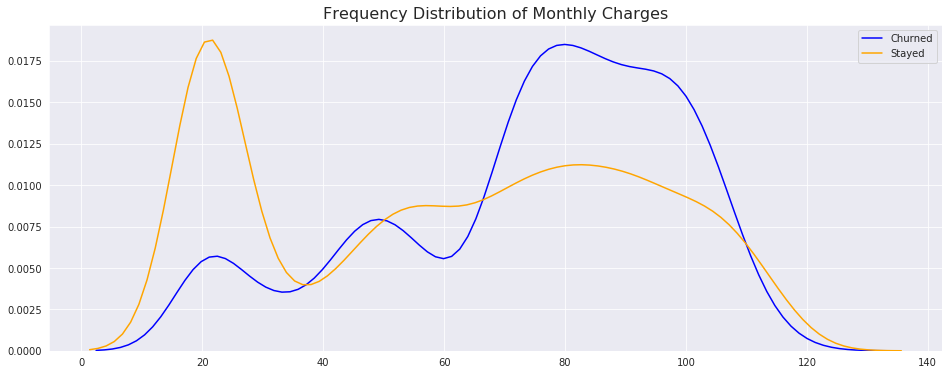``Since the correlation of monthly charges and tenure is weaker than that in the above case, the results pertain to the obvious ie people with higher monthly charges churned more``

#### Tenure vs Age group

In :
``````plt.figure(figsize=(14,8))
plt.title("Tenure vs Age group", fontsize = 16)
sns.stripplot(x="SeniorCitizen", y="tenure", data=dataset[dataset['Churn']=='Yes'],palette='Set2')
plt.show()
``````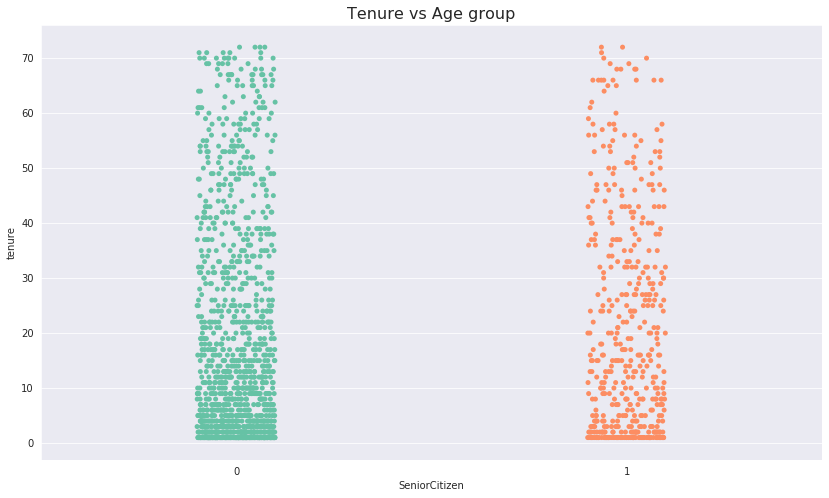``Since tenure and age (linear) are a functions of time, we compare these two linked variables. Looking at the almost identical scattering we can say being young or not was nowhere linked to a longer tenure (and thus a lower churn rate )``
In :
``````sns.catplot(x='Churn', y='tenure',data=dataset)
# plt.show()``````
Out:
``<seaborn.axisgrid.FacetGrid at 0x7f0f28b65518>``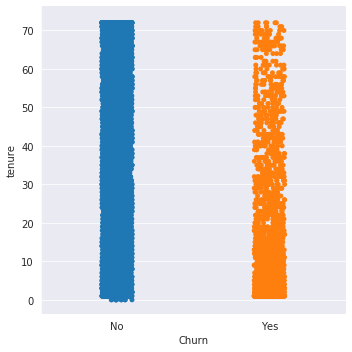``Further we can use a catplot to relate a categorical and a numerical feature. From the same we infer that as the tenure increases the density of ones who left reduces. Basically, older customers happened to stick around even more than the ones who left relatively early. ``
In :
``````plt.figure(figsize=(15,12))
plt.title('heat map!!11!!', fontsize = 18)
corr=dataset.apply(lambda x:pd.factorize(x)).corr()
sns.heatmap(corr,
xticklabels=corr.columns,
yticklabels=corr.columns,
linewidth=.5)
plt.show()
``````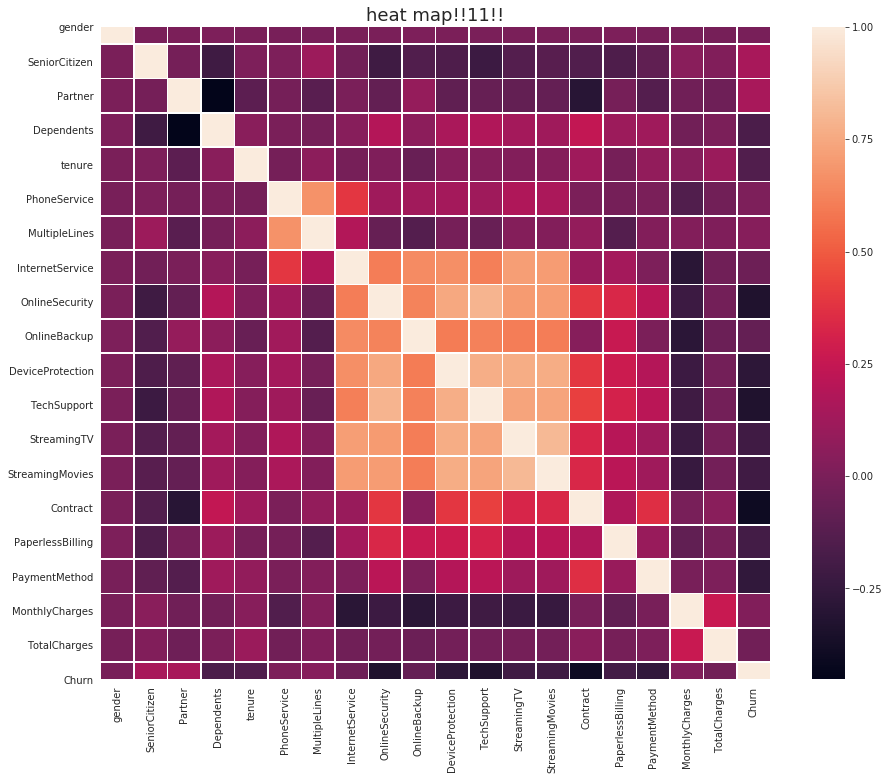``````The heatmap serves as a quick visualisation for correlation between all the features
``````

Note: the scale on the right is a an estimated metric for the 'strength of correlation'. The closer to 1 the stronger the two features are related

In [ ]:
``jovian.commit()``